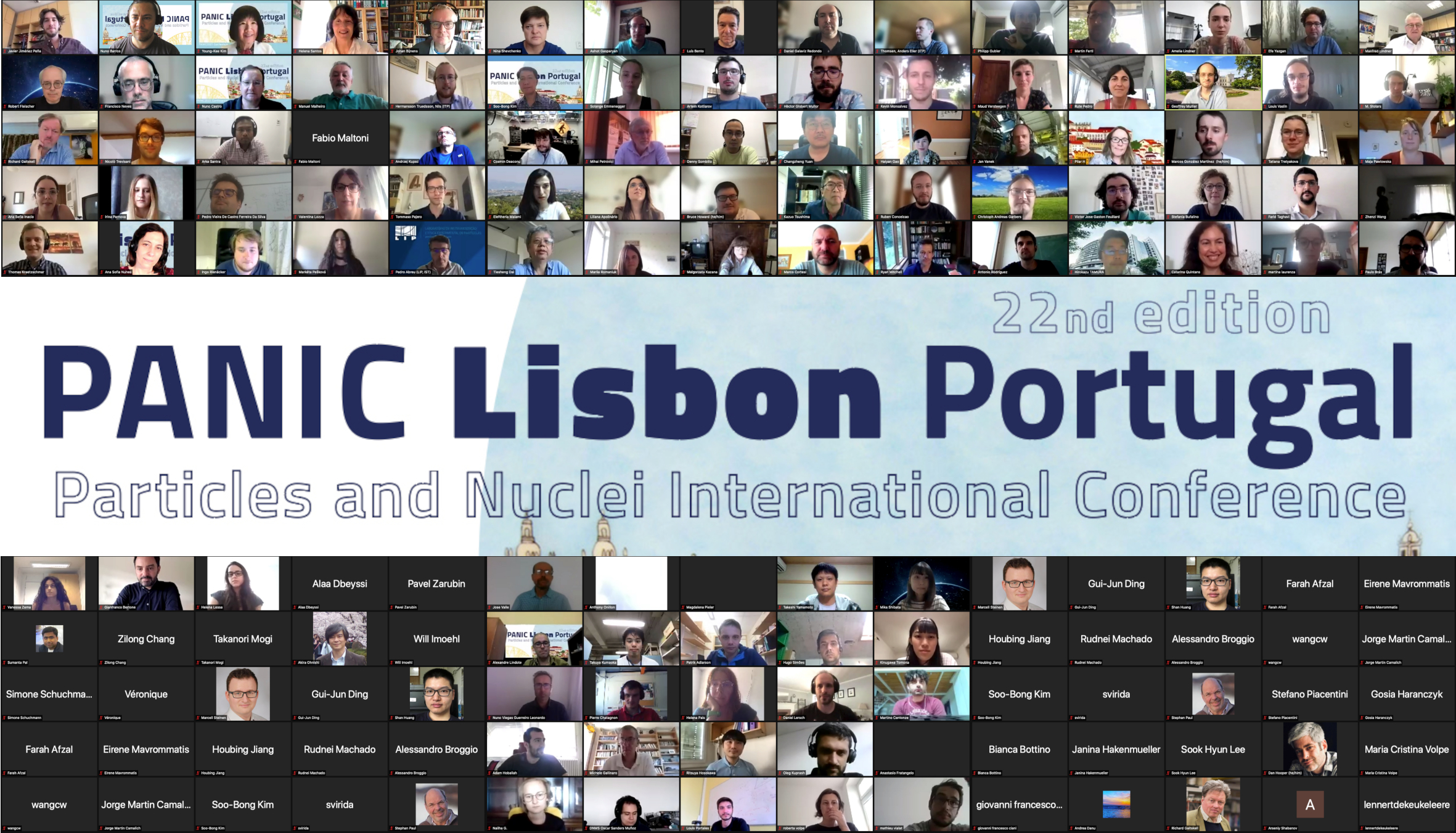#PANIC2021 Conference

Sep 5 – 10, 2021
Online
Europe/Lisbon timezone

## Systematic study of energy loss in quark-gluon plasma at RHIC-PHENIX

Sep 5, 2021, 4:02 PM
18m
Online

#### Online

Talk Hot and dense matter physics - QGP and heavy ion collisions

### Speaker

Ms Mika Shibata (Nara Women's University)

### Description

The phase transition from hadronic material to quark-gluon plasma (QGP) is a phenomenon that occurs under extreme conditions of high temperature and high density. The QGP causes energy loss of high momentum particles which is observed as a suppression of high momentum hadron production in A+A collisions relative to p+p collisions. PHENIX, one of the relativistic heavy ion collider (RHIC) experiments at Brookhaven National Laboratory, aims to measure various QGP signals from nuclear collision reactions. The study presented in this talk uses PHENIX data to evaluate the energy loss of partons in QGP in various collision systems. We systematically study the energy loss with $\pi^0s$ in Au+Au and Cu+Au and Cu+Cu at $\sqrt{s_{NN}}$=200 GeV and charged hadrons in Au+Au at $\sqrt{s_{NN}}$=200 GeV using two quantities, $S_{loss}$ and $dp_T$. $S_{loss}$ represents the percentage of high $p_T$ hadron momentum loss. Previous studies revealed the the scaling properties of $S_{loss}$ on collision system size. The current study uses $S_{loss}$ to quantify energy loss dependence on path-length and measures $dp_T$ which is the difference in momentum loss between the in-plane and out-of-plane directions.
The interpretation of these results and their impact on our understanding of the path-length dependence of energy loss in the QGP will be discussed.

### Primary author

Ms Mika Shibata (Nara Women's University)